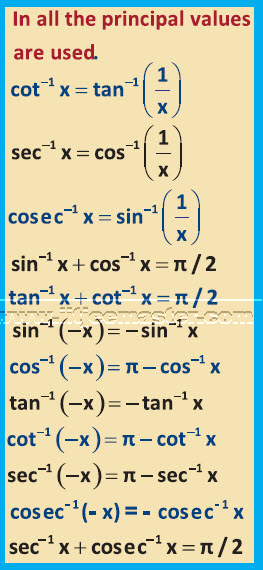IIT JEE video maths lectures will provide you illustrative examples for understanding the concepts better. In this video, lecture we will learn about yet important JEE mathematics topic of IIT-JEE examination. This jee maths video lecture is based on inverse trigonometric function.

It is important for us to know what inverse function is before understanding inverse trigonometric function. There are six trigonometric functions such as sin x, cos x, tan x, cot x, sec x, cosec x. You must have studied the concepts and functions of functions in class 12th itself. However, these video lectures will take you a step forward in understanding.

In 12th standard we must have learned that if the value of x varies, then value of sin x will also vary accordingly. Sin x will be said as the function of x, denoted by f(x). This entire process/ action is known as function of x. Hence, sin is the function and x is the variable here. X is the variable angle value.

Inverse function concept states that if there is a function g(x) such that g((f(x)) = x or f (g(x)) = x, then g(x) is inverse function of f(x). Hence if x is the angle then, sin x will be the ratio of sides. In the JEE topic also you need to understand the concepts very well. All the questions in the examination will be based on the concepts. These video lectures will help you to understand the concepts in simple manner.

Sin is an action on a variable. This implies that sin can be located with any number. Similarly sin inverse [sin -1 (x)] is also an action and can be performed on any number. Here also note that

[sin -1 (x)] is not 1/ sin x.  It is only a notation which denotes the inverse function of sin and nothing else.

In this way we can define six inverse trigonometric functions. Inverse of a function exists if and only if the function is one and onto. This is the most important concept of this chapter. Students often get confused to understand the function of one and onto.

Understanding each and every concept is important here to take you a step further in your IIT-JEE maths examination preparations.